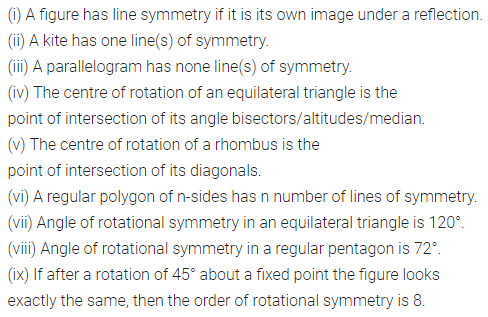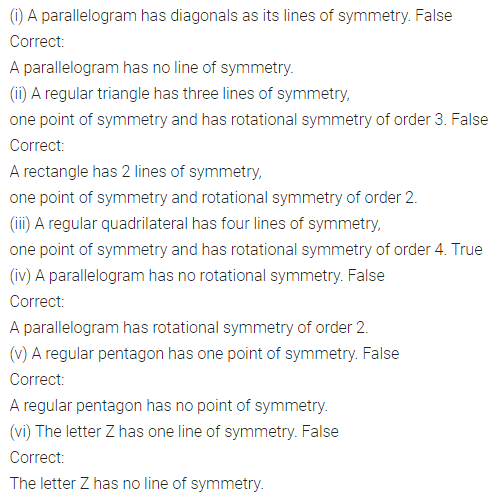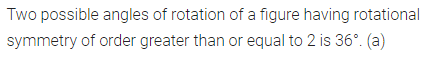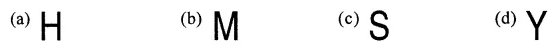# ML Aggarwal Class 8 Solutions for ICSE Maths Chapter 16 Symmetry Reflection and Rotation Objective Type Questions

## ML Aggarwal Class 8 Solutions for ICSE Maths Chapter 16 Symmetry Reflection and Rotation Objective Type Questions

Mental Maths
Question 1.
Fill in the blanks:
(i) A figure has ………….. symmetry if it is its own image under a reflection.
(ii) A kite has ………….. line(s) of symmetry.
(iii) A parallelogram has ………….. line(s) of symmetry.
(iv) The centre of rotation of an equilateral triangle is the point of intersection of its …………..
(v) The centre of rotation of a rhombus is the point …………..
(vi) A regular polygon of n-sides has ………….. number of lines of symmetry.
(vii) Angle of rotational symmetry in an equilateral triangle is …………..
(viii) Angle of rotational symmetry in a regular pentagon is …………..
(ix) If after a rotation of 45° about a fixed point the figure looks exactly the same, then the order of rotational symmetry is …………..
Solution:Question 2.
State whether the following statements are true (T) or false (F):
(i) A parallelogram has diagonals as its lines of symmetry.
(ii) A regular triangle has three lines of symmetry, one point of symmetry and has rotational symmetry of order 3.
(iiii) A, regular quadrilateral has four lines of symmetry, one point of symmetry and has . rotational symmetry of order 4.
(iv) A parallelogram has no rotational symmetry.
(v) A regular pentagon has one point of symmetry.
(vi) The letter Z has one line of symmetry.
Solution:Multiple Choice Questions
Choose the correct answer from the given four options (3 to 6):
Question 3.
The number of lines of symmetry which a quadrilateral cannot have
(a) 1
(b) 2
(c) 3
(d) 4
Solution:Question 4.
A possible angle of rotation of a figure having rotational symmetry of order greater than or equal to 2 is
(a) 36°
(b) 144°
(c) 150°
(d) 360°
Solution:Question 5.
The figure which does not have both line and rotational symmetry isSolution:Question 6.
The letter which has both line and rotational symmetry isSolution: